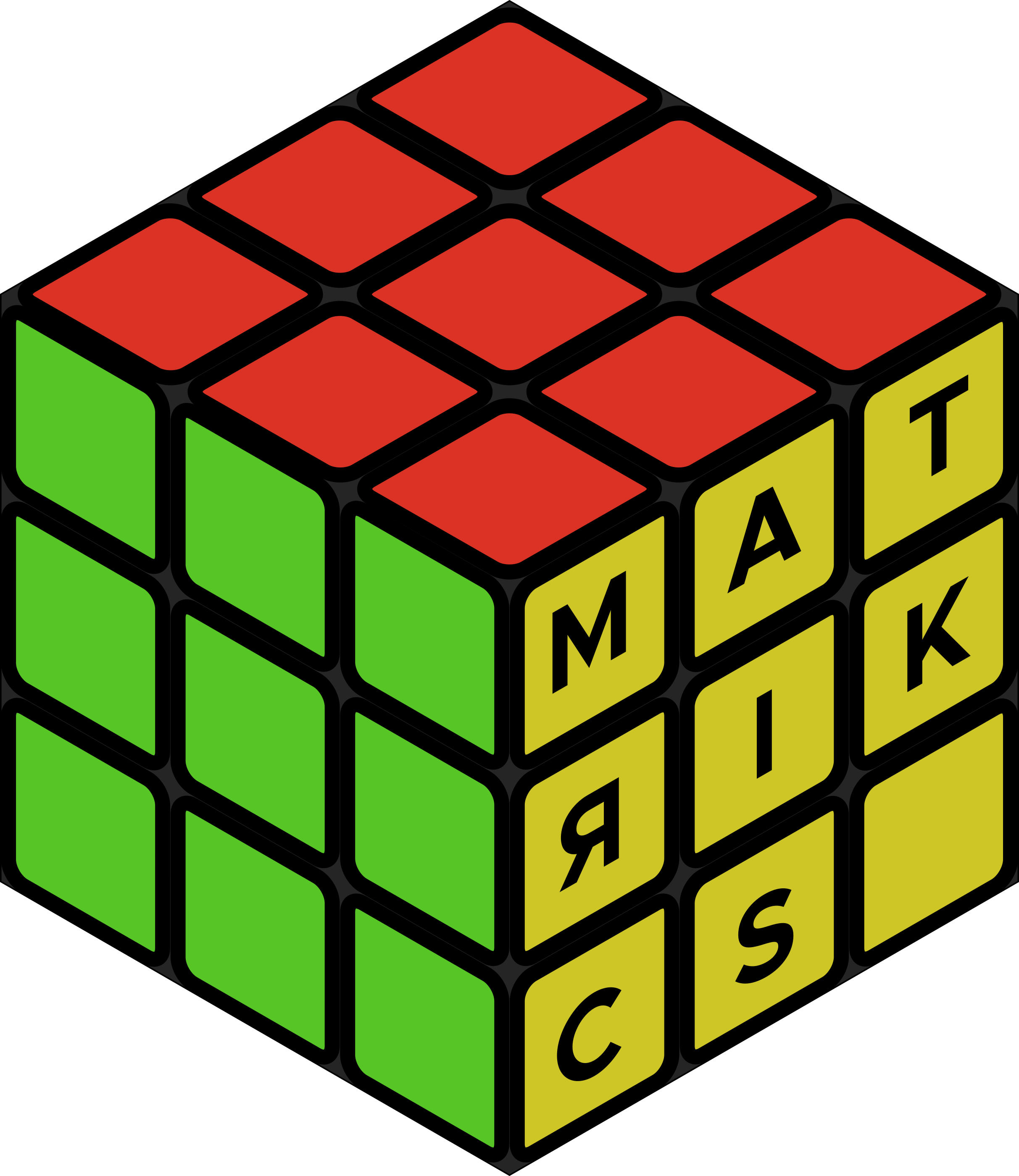# matricksUseful tricks for matrix manipulation

### Installation

``devtools::install_github('krzjoa/matricks')``

### Usage

Main `matricks` functions are `m` and `v`, which provide convenient API to create matrices and vectors.
Why should we write:

``````matrix(c(5, 6, 7,
8, 0, 9,
3, 7, 1), nrow = 3, byrow = TRUE)
#>      [,1] [,2] [,3]
#> [1,]    5    6    7
#> [2,]    8    0    9
#> [3,]    3    7    1``````

if we can simply create such a matrix like that:

``````library(matricks)

m(5, 6, 7|
8, 0, 9|
3, 7, 1)
#>      [,1] [,2] [,3]
#> [1,]    5    6    7
#> [2,]    8    0    9
#> [3,]    3    7    1``````

`v` function is an useful shortcut for creating vertical vectors (single columns)

``````v(1,2,3)
#>      [,1]
#> [1,]    1
#> [2,]    2
#> [3,]    3
v(1:5)
#>      [,1]
#> [1,]    1
#> [2,]    2
#> [3,]    3
#> [4,]    4
#> [5,]    5``````

Setting values in easier with `matricks`

``````mat <- matrix(0, 3, 3)
set_values(mat, c(1, 2) ~ 0.5, c(3, 1) ~ 7)
#>      [,1] [,2] [,3]
#> [1,]    0  0.5    0
#> [2,]    0  0.0    0
#> [3,]    7  0.0    0``````